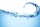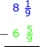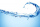# Mixed fractions calculator

The calculator performs basic and advanced operations with mixed numbers, fractions, integers, decimals. Mixed fractions are also called mixed numbers. A mixed fraction is a whole number and a proper fraction combined, i.e. one and three-quarters. The calculator evaluates the expression or solves the equation with step-by-step calculation progress information. Solve problems with two or more mixed numbers fractions in one expression.

## Result:

### 4 - 2/5 = 18/5 = 3 3/5 = 3.6

Spelled result in words is three and three fifths (or eighteen fifths).

### Calculation steps

1. Subtract: 4 - 2/5 = 4/1 - 2/5 = 4 · 5/1 · 5 - 2/5 = 20/5 - 2/5 = 20 - 2/5 = 18/5
For adding, subtracting, and comparing fractions, it is suitable to adjust both fractions to a common (equal, identical) denominator. The common denominator you can calculate as the least common multiple of both denominators - LCM(1, 5) = 5. In practice, it is enough to find the common denominator (not necessarily the lowest) by multiplying the denominators: 1 × 5 = 5. In the following intermediate step, the fraction result cannot be further simplified by canceling.
In other words - four minus two fifths = eighteen fifths.

#### Examples:

sum of two mixed numbers: 1 3/4 + 2 3/8
addition of three mixed numbers: 1 3/8 + 6 11/13 + 5 7/8
addition of two mixed numbers: 2 1/2 + 4 2/3
subtracting two mixed numbers: 7 1/2 - 5 3/4
multiplication of mixed numbers: 3 3/4 * 2 2/5
comparing mixed numbers: 3 1/4 2 1/3
changing improper fraction to mixed number: 9/4
What is 3/4 as a mixed number: 3/4
subtracting mixed number and fraction: 1 3/5 - 5/6
sum mixed number and an improper fraction: 1 3/5 + 11/5

## Mixed fractions in word problems:

• SubtractSubtract two mixed numbers: 3 1/2 -1 3/4
• Equation with mixed 2A number, X, is subtracted from 8 1/4. The result is 12 3/5. What is the value of X?
• Sum of 20Sum of the two numbers is 15 1/6. If one of the numbers is 2 2/3, find the other number.
• Chin swimChin swim 9 3/4 miles a week so far this week he was swum 3 1/4 miles. How many more miles does Chin need to swim this week?
• Mixed numbersFive and two-thirds minus 2 and one-half equals what number? A three and one-sixth B three and two-thirds C three and one-half D three and five-sixths
• 5 2/55 2/5 hours a week  mathematics,  3 3/4 hours a week   Natural sciences, 4 3/8 hours a week  Technology . how many hours does  he spend on social sciences if he spend 17 1/2 hours a week for the four subject?
• Fractions and mixed numerals(a) Convert the following mixed numbers to improper fractions. i. 3 5/8 ii. 7 7/6 (b) Convert the following improper fraction to a mixed number. i. 13/4 ii. 78/5 (c) Simplify these fractions to their lowest terms. i. 36/42 ii. 27/45 2. evaluate the follow
• Jose studiedJose studied for 4 and 1/2 hours on Saturday and another 6 and 1/4 hours on Sunday. How many subjects did he study if he has alloted 1 and 1/2 hours per subject on Saturday and 1 and 1/4 hours per subject on Sunday?
• BenhurBenhur boiled 1 1/4 liters of water in a kettle. After 10 1/2 minutes he measured the water again. It had 3/4 liters left in the kettle. What is the amount of water that evaporates every minutes?